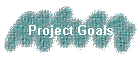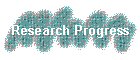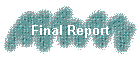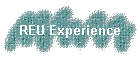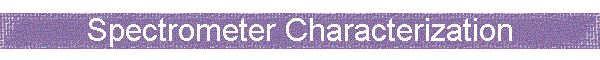Fair warning, this part is really sciency.  Have fun! :)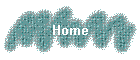Characterizing the EBIS-C Spectrometer in Nonfocusing and Focusing Modes

Goal:  Understand the performance of the EBIS-C spectrometer when used in different ways and determine the best operating conditions for both nonfocusing and focusing spectrometer modes.

The spectrometer characterized in this document consists of 27 equally spaced (5 mm apart) ring electrodes arranged according to the scale drawing shown below.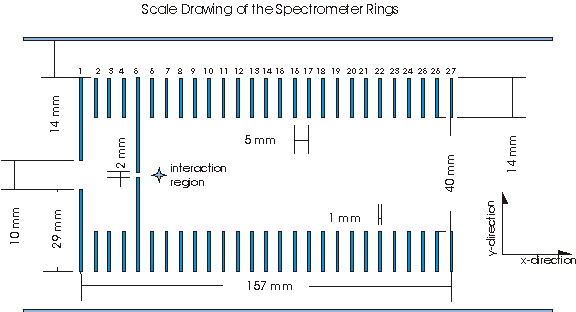Length of spectrometer:  157 mm

Length from end of spectrometer to detector:  696 mm

Length from interaction region to detector:  820 mm

Interaction region:  Midpoint between plates 6 and 7, which we choose to be xo = 57.5 mm and yo = 0 mm.

The rings themselves are 1mm thick stainless steel.  Plates 1-5 are connected by 100MΩ resistors.  Plates 5-27 are connected by 10MΩ resistors.  A grounded mesh is located 6 mm in front of the detector.

In this paper, the X direction is defined as parallel to the spectrometer axis (i.e. approximately the same direction that a beam travels through the spectrometer).  The Y direction is perpendicular to the spectrometer axis.  As the spectrometer has radial symmetry, this Y direction includes any possible angle in the plane perpendicular to the spectrometer.  Therefore, it will not be necessary to use a Z direction here.

Our analysis will consist largely of analyzing the time of flight (TOF) of particles under various conditions simulated by the SIMION program.  The TOF formula has the general form TOF = a(M/q)1/2 + To, where a and To­ ­are constants, M stands for the particle mass, and q is the particle charge.  This produces a linear relationship, which means that the TOFs of different particles under similar conditions are related by a factor of the square root of the mass to charge ratio.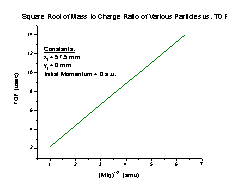Recoil Characteristics of Nonfocusing Spectrometer

The time of flight (TOF) of a recoil ion Argon (39.984 amu) when the spectrometer is used in nonfocusing mode with the spectrometer voltage (the voltage on the 5th plate) set at 1000 V, shows the following tendencies:

1.      The TOF increases linearly as the initial position of a particle along the X axis approaches the detector (Figure A). It follows the equation TOF = 12.29898 + 0.0296Xi, where Xi is the initial position of the particle.

2.  As the initial Y position of a particle gets farther from the spectrometer axis, the TOF increases in a manner we are not sure how to explain (Figure B).  It is most likely due to numerical limitations of SIMION.  At any rate, the effect is approximately 50 picoseconds in duration.  Our detector’s best practical time resolution is on the order of 50 picoseconds, so the effect is beyond our detector’s ability to measure.  Therefore, this should not affect the performance of the spectrometer.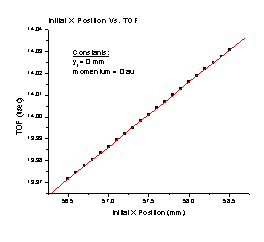Figure A                                                               Figure B

3.  As the initial momentum of the Ar+ particle parallel to the spectrometer axis increases (Figure C), the TOF decreases with a perfect correlation to the formula TOF = 14.00107 – 0.0016 p – (7.14132 x 10-7) p2, where p is the momentum in a.u.

4.  Increasing the initial momentum in the Y direction with no momentum in the X direction results in an increased TOF (Figure D).  It follows the equation TOF = 14.00105 + (3.23803 x 10-6) p + (9.17684 x 10-7) p2, where p is the initial momentum of the particle in a.u.  Note that this is a small effect.  This entire graph covers only 5 nanoseconds, and most of the structure occurs with differences of a few hundred picoseconds.  It is quite possible at this point that we are taxing SIMION to its limit.  The slight structure seen on this graph is a result of SIMION’s limitations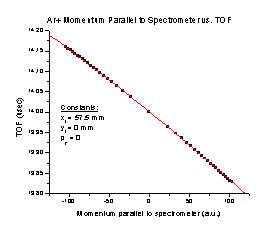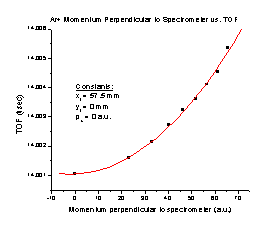Figure C                                                       Figure D

To test whether the TOF would behave in a similar fashion with a particle of different mass than Ar+, we tried some of the same runs again using H+ (1.008 a.m.u.).

To determine whether the TOF would behave in a similar fashion, we compared the TOF equations we found for the two particles.  Since the general form of the equations is a constant times (M/q)1/2, plus To that is determined only by our equipment, the equations should be similar by a factor of square root M/q, where M is the mass of the particle and q is the charge.  So to compare H+ and Ar+ TOFs, we divide the Ar+ TOF by the H+ TOF.  Since both particles have a charge of one, if the equations are similar we should get the square root of the particles’ mass ratio (6.29531).

1.  The TOF increases linearly as the initial position of H+ along the X axis approaches the detector (Figure E). It follows the equation TOF = 1.95364 + 0.0047Xi, where Xi is the initial position of the particle.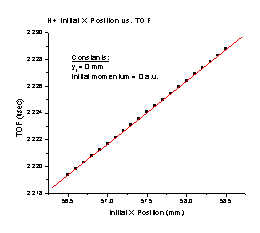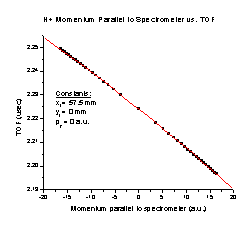Figure E                                                   Figure F

 Xi Value (mm) H+ TOF (µsec) Ar+ TOF (µsec) TOF Ar+/ TOF H+ 0 1.95364 12.29898 6.29542 1 1.95834 12.32858 6.29542 2 1.96304 12.35818 6.29543 3 1.96774 12.38778 6.29544

The best practical time resolution of our detector is on the order of 50 picoseconds.  This means that the TOF Ar+/TOF H+ column is sufficiently equal, because we will only be able to measure accurately to the 4th decimal place.  The range of values displayed is sufficient to affirm that the equations are similar, because the initial X position should not be greater than 2 mm.  Therefore, we conclude that the initial X position affects the TOF in the same way without dependence on the mass of the particle, although we are not sure why the TOF Ar+/TOF H+ value we found is not quite the same as the mass ratio.

2.  As the initial momentum of the H+ particle parallel to the spectrometer axis increases (Figure F), the TOF decreases with a perfect correlation to the formula TOF = 2.22405 – 0.0016 p – (4.47326 x 10-6) p2, where p is the momentum in a.u.

 Pix Value (a.u.) H+ TOF (µsec) Ar+ TOF (µsec) TOF Ar+/TOF H+ 0 2.22405 14.00107 6.29530 10 2.20805 13.98500 6.33364 50 2.13287 13.91928 6.52608

This shows that the initial momentum of a particle in the X direction does not affect the TOF in the same way for particles of different masses

3.  Increasing the initial momentum of H+ in the Y direction with the momentum in the X direction at zero results in an increased TOF (Figure G).  It follows the equation TOF = 2.22405 + (3.44398 x 10-6) p + (5.75993 x 10-6) p2, where p is the initial momentum of the particle in a.u.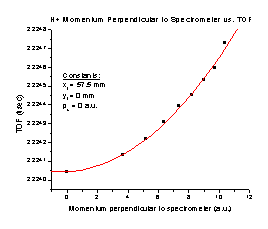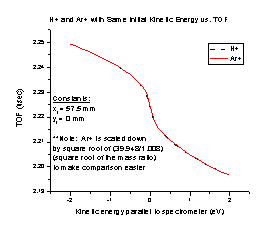Figure G                                                      Figure H

 Piy Value (a.u.) H+ TOF (µsec) Ar+ TOF (µsec) TOF Ar+/TOF H+ 0 2.22405 14.00105 6.29529 10 2.22466 14.00117 6.29362 20 2.22642 14.00148 6.28879

The initial momentum of a particle in the Y direction does not affect the TOF in the same way for particles of different masses.

We also tested the spectrometer with two particles of different mass but the same kinetic energy (Figure H).  We expect the particles in an electrostatic device such as our spectrometer to follow the ratio E/q, where E is the energy of the particle and q is its charge.  Therefore, the two graphs should be different only by a factor of the square root of m/q.  To check this we divided the Ar+ TOF values by the square root of the mass ratio (Ar+/H+), using the particle masses we entered in SIMION (39.948 amu and 1.008 amu, respectively).  The charge is already included in that calculation, since both particles have a charge of one.  With proper scaling by the square root of the mass ratio, particles with the same kinetic energy have the exact same TOF.  This means that particles in the spectrometer do follow the ratio E/q.

We also tried the spectrometer with two particles that have the same momentum but different masses (Figure I), again scaling the Ar+ graph by the square root of the mass ratio.  It makes sense that when the initial momentum is zero the scaled TOF is the same, based on our earlier analysis of the H+ initial X position graph.  As the momentum increases, the velocity for the H+ particle increases much faster than the velocity of the Ar+ particle, so the H+ TOF should decrease much more quickly than that of Ar+, as the graph shows.  However, this means that we will have to find equations for each individual particle when we are calculating momentum.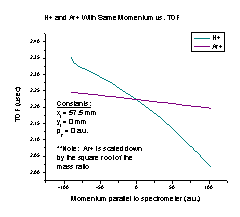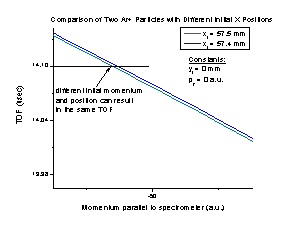Figure I                                                       Figure J

Using the spectrometer in nonfocusing mode will cause problems when calculating a particle’s initial momentum in certain experiments.  We calculate the initial momentum of a particle from the TOF and using momentum conservation.  However, it is possible for particles with different initial positions and momenta to have the same TOF, as shown in Figure J of a particle starting at 57.5 mm (in green) and at 57.4 mm (in blue).

It is possible for a particle with any given initial momentum and position to share a common time of flight with another particle that has the correct different initial momentum and position.  Therefore, in order to calculate the initial momentum properly, sometimes it will be necessary to use the spectrometer with either space or time focusing.

Recoil Characteristics of Focusing Spectrometer

Operating the spectrometer in focusing mode requires very few modifications.  Instead of allowing the voltage to decrease linearly from plates 5-27 as it does in nonfocusing mode, a focusing voltage (Vf) is placed on the 11th plate of the spectrometer.  Then the voltage decrease is as shown in Figure K (exact voltage values will vary based on the Vf voltage used):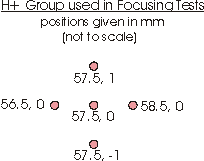Figure K                                                              Figure L

In the interest of keeping the following analysis applicable to any Vs the spectrometer is run at, we will think of the Vf as a particular percent of the Vs.  However, in order to keep our experiments uniform for comparison, we will use Vs = 1000 V.

To find the Vf that works best for the EBIS-C spectrometer using SIMION, we set the Vs at 1000 V, varied the percentage of the Vs that we used for the Vf, and flew a group of H+ particles (Figure L) starting from rest at the center of the interaction region through the spectrometer.  The group consisted of five particles with initial (x,y) positions of   (56.5, 0), (57.5, 0), (58.5, 0), (57.5, -1), and (57.5, 1) mm respectively.

Time Focusing

Applying a Vf to the spectrometer allows particles with different initial momenta to “catch up” to each other.  A perfectly time focused spectrometer would cause any two particles that started at the same time to hit the detector at the exact same time, no matter what their respective initial momenta were.

To find the best time focusing for the spectrometer (Figure M), we varied the Vf  while flying the H+ group using the conditions described above.  For each Vf trial, SIMION reports the ending TOF of each individual particle.  From that, we found the highest and lowest TOFs of the group.  We subtracted the lowest from the highest and divided the result by two, which gave us the error in the time focusing when using that particular Vf.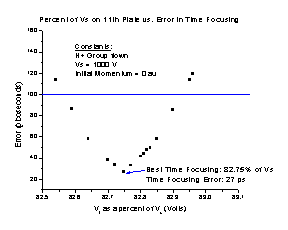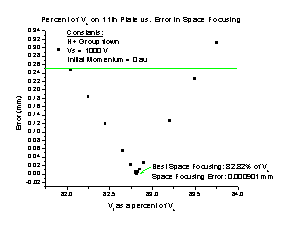Figure M                                                          Figure N

The best operating time focusing voltage is 82.75% of the Vs.  However, as we need to determine the best Vf to achieve space focusing as well, it should be noted that any Vf between the range of 82.59% and 82.95% will easily keep the error less than 100 picoseconds.

Space Focusing

A perfectly space focused spectrometer would cause all particles to hit the detector at the same spot, regardless of their initial position.  To find the best space focusing for the spectrometer (Figure N), we varied the Vf  while flying the H+ group using the conditions described above.  For each Vf trial, SIMION reports the ending Y positions of each individual particle.  From that, we found the highest and lowest Y positions of the group.  We subtracted the lowest from the highest and divided the result by two, which gave us the error in the space focusing when using that particular Vf.

The best operating space focusing voltage is 82.82% of the Vs, with an error of 0.0009 mm.  However, the resolution of our detector is only 0.25 mm, so any Vf that results in an error less than that will give us perfect space focusing, as far as our experimental capabilities are concerned.

Best Overall Operating Voltage

If the spectrometer is operated at the optimum space focusing voltage, the time focusing error will be 47.5 ps, which is still below the best practical resolution of the detector.  If the spectrometer is operated at the optimum time focusing voltage, the error in space focusing will be 0.022 mm, also well below the resolution of our spectrometer.  Since equipment that will allow the Vf percentage to be accurate to two decimal places is very expensive, it should be noted that operating the spectrometer at 82.8% will give the best overall operating conditions for the spectrometer.  It contains the best time focusing voltage within the range of rounding to one decimal place, and even if the actual Vf goes as far off as 82.85%, the largest time focusing error would be 58 ps.  The largest space focusing error would be .022 mm, still way below the resolution of our detector.

 Vf Largest Time Focusing Error (ps) Largest Space Focusing Error (mm) 82.6% 113 0.09 82.7% 58 0.06 82.8% 58 0.02 82.9% 113.5 0.06

We tested the accuracy of the Vf for particles of different mass to see if the resolution would still act as expected.  The space focusing is not affected by particles of different mass, because after testing groups of H+, C+, and Ar+ particles in the spectrometer with Vf = 82.8%, we found that all three groups had the same space focusing error.

 Particle Group Space Focusing Error (mm) H+ 0.0057 C+ 0.0057 Ar+ 0.0057

The time focusing error is also the same for particles of different masses, but to test it we needed to look at the relative time error for different particle groups flown with Vf = 82.8%. To find the relative time focusing error, we divided the time focusing error of the particle group by the lowest TOF in the group.

 Particle Group Relative Time Focusing Error (Error/TOF) H+ 18 x 10-6 C+ 18 x 10-6 Ar+ 18 x 10-6

The error in the focusing is about the same for any Vs.   With the Vs at different levels and the Vf always at 82.8%, we flew the H+ group and found very similar relative time focusing errors.    The difference between them is so small that we can just ignore it and say the time focusing is equally good when the spectrometer is set at any Vs.  It is not necessary to run the space focusing tests as well, since the space focusing error is so far below the resolution of our detector.

 Spectrometer Voltage Relative Time Focusing Error 500 V 20 x 10-6 1000 V 18 x 10-6 2000 V 17 x 10-6 5000 V 15 x 10-6

As several intended experiments with this spectrometer place emphasis on the time focusing of Ar+, its actual time focusing error is given below.  It should be noted that for Ar+ from a time focusing standpoint, 82.7% is a better Vf.  So, as ions get heavier, if the main goal of the experiment is time focusing, the spectrometer should be operated at 82.7%.

 Vf Time Focusing Error (ps) Space Focusing Error (mm) 82.6% 505.5 0.07086 82.7% 242.5 0.03812 82.8% 261 0.00565 82.9% 538.5 0.02714

Conclusion

For a basic focusing spectrometer operating summary, remember

1.  To best focus the spectrometer, the Vf should be at 82.8% of the Vs.

2.  The spectrometer focuses equally well at any Vs.

3.  The spectrometer focuses all size particles equally well.

This spectrometer has excellent time and space focusing capabilities and should work well for a range of different experiments.